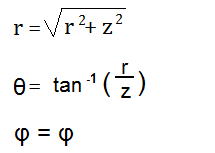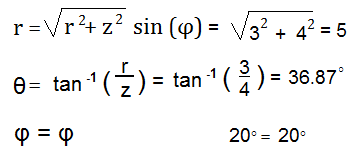﻿ Cylindrical to Spherical Coordinates Calculator ﻿# Cylindrical to Spherical Coordinates Calculator### Cylindrical to Spherical Coordinate System DiagramThis spherical coordinates converter/calculator converts the cylindrical coordinates of a unit to its equivalent value in spherical coordinates, according to the formulas shown above.

Cylindrical coordinates are depicted by 3 values, (r, φ, Z). When converted into spherical coordinates, the new values will be depicted as (r, θ, φ).

To use this calculator, a user just enters in the (r, φ, z) values of the cylindrical coordinates and then clicks 'Calculate', and the spherical coordinates will be automatically computed and shown below.

By default, the calculator will compute the result in degrees. However, by using the drop-down menu, the option can changed to radians, so that the result will be computed in radians.

Example Calculation

Convert the cylindrical coordinates (3, 20°, 4) into its equivalent spherical coordinates.This answer is calculated in degrees.

Related Resources

﻿